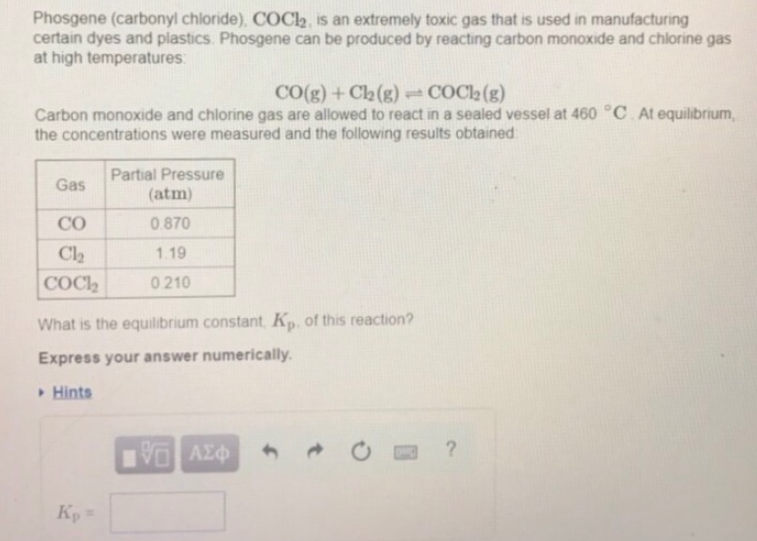Chemistry Practice Problems Equilibrium Expressions Practice Problems Solution: Phosgene (carbonyl chloride), COCl2, is an extreme...

🤓 Based on our data, we think this question is relevant for Professor Hayden's class at OAKLAND.

# Solution: Phosgene (carbonyl chloride), COCl2, is an extremely toxic gas that is used in manufacturing certain dyes and plastics. Phosgene can be produced by reacting carbon monoxide and chlorine gas at high temperatures: CO(g) + Cl2(g) ⇌ COCl2(g) Carbon monoxide and chlorine gas are allowed to react in a sealed vessel at 460°C. At equilibrium, the concentrations were measured and the following results obtained: What is the equilibrium constant, Kp of this reaction? Express your answer numerically.

###### Problem

Phosgene (carbonyl chloride), COCl2, is an extremely toxic gas that is used in manufacturing certain dyes and plastics. Phosgene can be produced by reacting carbon monoxide and chlorine gas at high temperatures:

CO(g) + Cl2(g) ⇌ COCl2(g)

Carbon monoxide and chlorine gas are allowed to react in a sealed vessel at 460°C. At equilibrium, the concentrations were measured and the following results obtained:

What is the equilibrium constant, Kp of this reaction? Express your answer numerically.View Complete Written Solution

Equilibrium Expressions

Equilibrium Expressions

#### Q. For the reaction shown here 2NH3 (g) ⇌ 3 H2 (g) + N2 (g) the equilibrium concentrations were found to be [NH3] = 0.250 M [H2] = 0.540 M [N2] = 0.800 M...

Solved • Fri Sep 07 2018 12:21:22 GMT-0400 (EDT)

Equilibrium Expressions

#### Q. Calculate the value of Kp for the equation C (s) + CO2 (g) ⇌ 2CO (g)                       Kp = ? given that at a certain temperature C (s) + 2H2O (g)...

Solved • Thu Sep 06 2018 17:20:27 GMT-0400 (EDT)

Equilibrium Expressions

#### Q. Write the following equilibrium-constant expressions: (a) Kc for Cr (s) + 3Ag+ (aq) ⇌ Cr3+ (aq) + 3Ag (s), (b) Kp for 3Fe (s) + 4H2O(g) ⇌ Fe3O4 (s) + ...

Solved • Wed Sep 05 2018 17:19:58 GMT-0400 (EDT)

Equilibrium Expressions

#### Q. Consider the following elementary reaction: ICl (g) + H2 (g) → HI (g) + HCl (g) Suppose we let k1 stand for the rate constant of this reaction, and k-...

Solved • Wed Sep 05 2018 11:31:22 GMT-0400 (EDT)